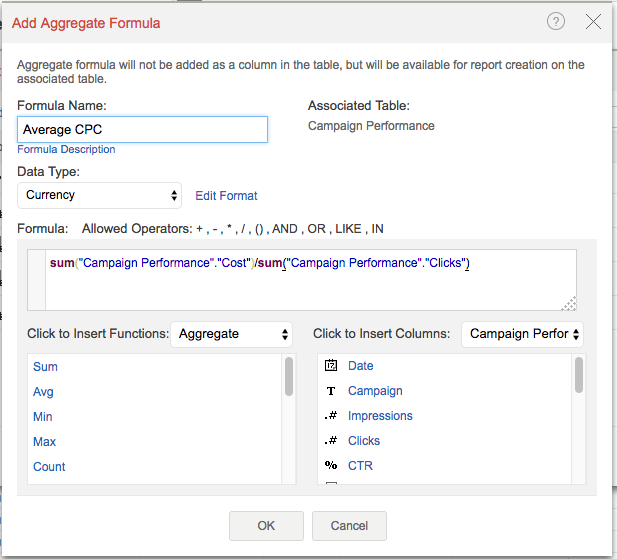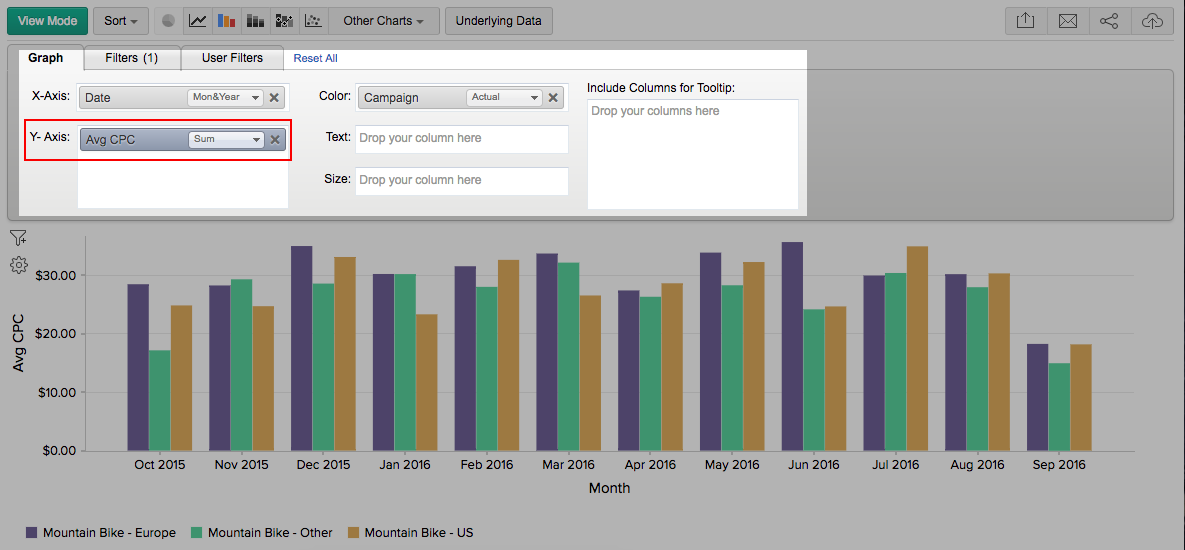# More Power to the Math Wizard in You – Aggregate Formulas

Zoho Analytics has long supported custom formulas, where you can define your own formula that involves two or more columns. These formulas are applied at the row level. Say there is Column A and Column B and you want a Column C which does string concatenation (combining texts). You can have a formula column defined as

concat(Column A, Column B).

There are scenarios where aggregation formulas (sum, avg, min, max etc) are to be applied at the column level. Here’s a real world example. In Google AdWords, Click Through Rate (CTR) is defined as (No. of Clicks)/(No. of Impressions). If you just go by averaging the CTR as Avg(CTR), you will end up with the wrong average value for the month, quarter or year. But if you let Zoho Analytics know that CTR is actually sum(Clicks)/sum(Impressions) by using the Aggregate Formula, then you’ll arrive at the right values.

You can add an aggregate formula by using Add -> Aggregate Formula option in a table’s toolbar.When creating reports (charts, pivot tables, summary views), you can use these aggregate formulas as you would use the regular columns or custom formula columns.Can you think of other scenarios where you can use the power of Aggregate Formulas in Zoho Analytics? Let us know in the comments.